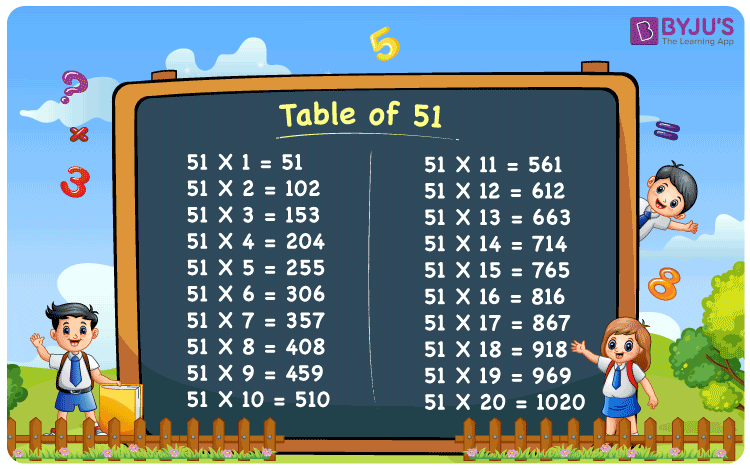# Table of 51

The Table of 51 is given here to help the students to learn the 51 times table up to 20. Learning the multiplication table of 51 will help you in numerical simplifications that involve the multiples of 51. In this article, you will get the image of the 51 times table, chart and a downloadable PDF of the table of 51, to understand and learn the multiplication table of 51 quickly.

## Table of 51 Chart

The chart given below will help you to memorize the 51 times table up to 120.## What is the 51 times table?

As we know, Maths tables can be written using different techniques such as addition and multiplication. The below table shows how we can write the 51 times table using multiplication and repeated addition of the number 51.

 Using multiplication Using repeated addition 51 × 1 = 51 51 51 × 2 = 102 51 + 51 = 102 51 × 3 = 153 51 + 51 + 51 = 153 51 × 4 = 204 51 + 51 + 51 + 51 = 204 51 × 5 = 255 51 + 51 + 51 + 51 + 51 = 255 51 × 6 = 306 51 + 51 + 51 + 51 + 51 + 51 = 306 51 × 7 = 357 51 + 51 + 51 + 51 + 51 + 51 + 51 = 357 51 × 8 = 408 51 + 51 + 51 + 51 + 51 + 51 + 51 + 51 = 408 51 × 9 = 459 51 + 51 + 51 + 51 + 51 + 51 + 51 + 51 + 51 = 459 51 × 10 = 510 51 + 51 + 51 + 51 + 51 + 51 + 51 + 51 + 51 + 51 = 510

## Multiplication Table of 51

Go through the multiplication table of 51 up to 20 multiples given below.

 51 × 1 = 51 51 × 2 = 102 51 × 3 = 153 51 × 4 = 204 51 × 5 = 255 51 × 6 = 306 51 × 7 = 357 51 × 8 = 408 51 × 9 = 459 51 × 10 = 510 51 × 11 = 561 51 × 12 = 612 51 × 13 = 663 51 × 14 = 714 51 × 15 = 765 51 × 16 = 816 51 × 17 = 867 51 × 18 = 918 51 × 19 = 969 51 × 20 = 1020

## Examples on the Table of 51

Example 1:

Evaluate the following:

(i) 16 times 51 plus 25

(ii) 19th multiple of 51

Solution:

(i) 16 times 51 plus 25

= 16 × 51 + 25

Using the multiplication table of 51, 51 × 16 = 816.

= 816 + 25

= 841

(ii) 19th multiple of 51

We know that 19th multiple of 51 = 19 times 51

Using the table of 51, 51 × 19 = 969.

Therefore, the 19th multiple of 51 = 969.

Example 2:

Shyam walks 4 km every day in the morning. How much distance does he cover (for a morning walk) in 51 days?

Solution:

The distance covered by Shyam in one morning = 4 km

The distance covered by Shyam in 51 mornings = ?

Using the multiplication table of 51, 51 × 4 = 204

Therefore, Shaym covers a distance of 204 km in 51 days of morning walk.

## Frequently Asked Questions on Table of 51

### What is the table of 51?

The table of 51 is the multiplication table 51 that can be generated by multiplying 51 with integers 1, 2, 3, and so on. The table of 51 up to 10 times is given as: 51 × 1 = 51 51 × 2 = 102 51 × 3 = 153 51 × 4 = 204 51 × 5 = 255 51 × 6 = 306 51 × 7 = 357 51 × 8 = 408 51 × 9 = 459 51 × 10 = 510

### Is 51 in the 3 times table?

Yes, 51 appears in the 3 times table, i.e. 3 times 17 = 51. Alternatively, we can observe the results of the 3 times table, i.e. 3, 6, 9, 12, 15, 18, 21, 24, 27, 30, 33, 36, 39, 42, 45, 48, 51, 54, 57, 60,…

### What multiplication makes 51?

Multiplication of the following pairs makes 51. 1 × 51 = 51 3 × 17 = 51 Or 17 × 3 = 51 51 × 1 = 51

### In which table does 51 come as an answer?

In the 3 times table and 17 times table, we get the answer 51. This can be expressed as: 3 × 17 = 51; 3 times 17 17 × 3 = 51; 17 times 3